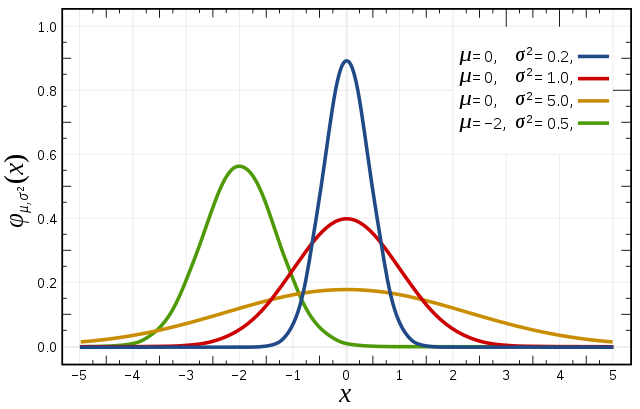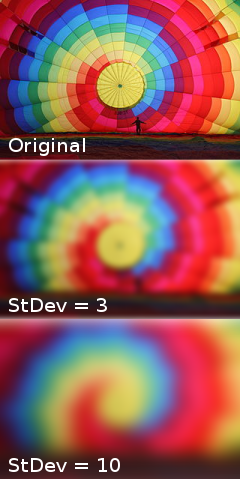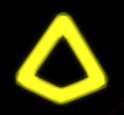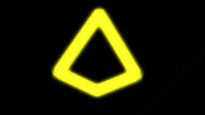# Blur under the lens

Welcome to the Positron Dev Log!

With most of the foundation ready, development seems much smoother now, with less work required to achieve what I want. This is specially good since I *am* starting to feel a little burnt out, so I won't be speeding up the development, but I think I will be able to "finish" the game before October, as planned!

For this week, I want to talk about the main shader I use for the game. While I'm still quite a noob, I will try to share the knowledge I was able to gather to make the blur effect, and hopefully this will be helpful or interesting to you somehow!

One common technique to blur an image is called the Gaussian blur. Plainly speaking, what it does is for each pixel on a image, it takes a number of neighboring pixels and calculates a weighed mean, according to the Gauss function/Bell curve/normal distribution.The effect it has in the image is, well, a blur, hiding noise and details, depending on the level of "blurriness" you choose:If you imagine an image  as a matrix of number (which, actually, it is) you would be multiplying the value of each pixels through this "mask" and dividing it with the sum of the "weights":

Now, with shaders like GSLS you don't actually get the grid of pixels, but you access each point of the image with UV coordinates. So to do that, I made the following loop:

```    for (int i = 1; i <= Steps; ++i) {
for (int arc = 0; arc < ArcsNumber; arc++) {
float teta = 360.0 / float(ArcsNumber) * float(arc);
vec2 texCoord = coord + vec2(cos(teta)  * resolution, sin(teta)) * float(i) / float(Steps) * blurRange * pixelSize;
float weight = blurWeight(float(i)/float(Steps));
total += weight * texture(tex, texCoord);
totalWeight += weight;
}
}```

(I'm using godot to make this game, and it uses it's own shading language, but it should be understandable anyway.)

So, let's explain first what are some of the variables that are not declared in the loop. Steps basically means how many steps should the shader take to calculate in between the center of the pixel to the furthest range (the blurRange), and that's what i. Similarly, ArcsNumber means how many arcs should we consider on the circumference around the center, and arc iterates through it. Since we access the texture pixels through UV coordinates, pixelSize is the size of a pixel in UV coordinates, in theory, but in the end I made it a uniform so I can change it to have different effects. texture(tex, texCoords) is fairly straight forward, it's the built-in function in godot to the the pixel from a texture on the determined coordinag

Lastly, we have the blurWeight function:

```float blurWeight(float x) {
return exp(-0.5 * (x * x) / (Sigma * Sigma));
}```

It's used to calculate what should be the weight of the pixel, according to the step it is in. It's an attempt to simulate the weighting of the Gaussian curve, but not a complete one, since it only takes into account the radius between the accessed pixel to the center, but it will do it for now. The result became like this:To give a little more life to the neon effect I played a little with the blur parameters and time, and the result was this pulsating effect:Again, not a super duper effect, but it's the subtle change that is enough to make it more interesting, that's what I usually like.

### Conclusion

In this post I tried to show how I managed to make a blur effect, and, even though it's not the Gauss blur by the book, I think it created an interesting effect. Some adaptations were required, but in the end, knowing the theory behind the technique, I was able to reproduce it to some level to use it in my game~.

### Last Words

I fell this one was a little shorter than usual, but since it's much more dense, I think it's better to leave it like this. If there is something that wasn't clear enough, or you want me to give more details for other techniques, feel free to ask me! For the next week I will be talking about how I came up with the theme music for the game! Stay tuned~